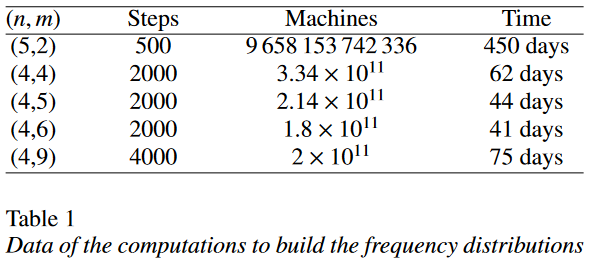Our story begins with a friend sending me this article from New Scientist.

The short version is, the authors gave a survey to several thousand people, asking them to generate random sequences. Then they measured how random those sequences were, and it turns out they were most random in 25 year olds, with a decline afterwards. It’s a neat result.

How is the randomness measured?

To measure how random people’s answers were, the researchers used a concept called “algorithmic randomness”. The idea is that if a sequence is truly random, it should be difficult to create an algorithm or computer program that can generate it. Statistics software provided an estimate of how complex this would be for each response.

Wait. What?

If you know a bit of complexity theory, this should set off alarm bells, because any problem of the form “Program $$P$$ has behavior $$B$$” tends to be a big ball of undecidable pain. (See Rice’s theorem, if interested.) Why does this study even use algorithmic randomness? How did complexity theory enter the picture?

* * *

To answer these questions, we need to dig to the original paper. Luckily, the full text is available online. A quick skim turns up this line.

An estimate of the algorithmic complexity of participants’ responses was computed using the acss function included in the freely publicly available acss R-package that implements the complexity methods used in this project.

Bingo! I’m guessing that either prior work used acss, or someone involved found out R had a library for measuring randomness. This still doesn’t answer what they’re doing for algorithmic complexity (which is decidedly, uh, undecidable.)

Following the citation for acss turns up the paper explaining the methodology. Turns out the acss paper shares authors with the New Scientist paper. Makes sense.

Now, let me set the stage for what happened next. I open this paper, and start rapidly scrolling through the pages. Then my eyes catch this passage.

To actually build the frequency distributions of strings with different numbers of symbols, we used a Turing machine simulator, written in C++, running on a supercomputer of middle-size at CICA.

Uhhhhhh. Turing machine simulator? On a supercomputer?

Then I read the table immediately after it, and my eyes kind of bug out.450 days of computing time? To simulate over 9 trillion Turing machines?

I’ve been going “Uhhhhh” this whole time. But on reading this table, I upgrade from “uhhhhh” to “UHHHHHH”.

But with a little more reading, I go from “UHHHHHH” to “YES”.

Gather round. It’s time to jump into complexity theory.

* * *

The goal of the library is to estimate the Kolmogorov complexity of various strings. In very rough terms, the Kolmogorov complexity is the length of the string’s shortest description. How is that connected to randomness? Intuitively, if you can describe a string $$x$$ quickly, it must have lots of structure, and therefore can’t be very random. If it’s hard to describe $$x$$, it must have little structure, which should count as very random. Therefore, we can define the randomness of sequence $$x$$ by its Kolmogorov complexity $$K(x)$$.

One problem: Kolmogorov complexity is undecidable. But we can reformulate Kolmogorov complexity into a form that can be approximated in a computable way. Turns out there’s a theorem (Levin, 1974) that relates Kolmogorov complexity to randomly generated Turing machines. (I was a bit surprised I didn’t know it already, to be honest.)

Consider a randomly sampled Turing machine, sampled such that programs of length $$\ell$$ are sampled proportionally to $$2^{-\ell}$$. Then

$K(x) = -\log_2 Pr(\text{random program outputs } x) + O(1)$

Where the constant $$O(1)$$ is independent of $$x$$.

In this form, Kolmogorov complexity is easy to approximate. We can simply sample a large number of random Turing machines, counting the fraction that outputs $$x$$.

And that’s exactly how the acss package was made! To estimate $$K(x)$$ for all $$x$$ of length at most $$11$$, they randomly generate a MASSIVE number of short Turing machines, run all of them, then accumulate them into probabilities for each $$x$$.

I mean, sometimes they run into pesky things like the halting problem, but they just pick a limit $$T$$ on the number of steps to run each machine, such that almost all of the machines halt, and it turns out a $$T$$ around $$4000$$ or so is good enough.

Well, there’s the story. In 2015, some people simulated trillions and trillions of Turing machines, saving the results into an R package. Then, they used it in a survey testing people’s ability to generate randomness, which finally turned into an article claiming that random number generation is a good proxy for cognitive ability.

I’m not sure I believe it’s a good proxy. But hey, that Turing machine thing was definitely cool.